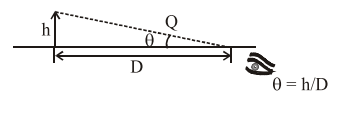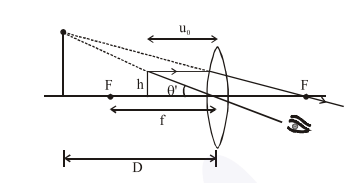# Given below are two statements : one is labelled as Assertion A and the other is labelled as Reason R.Question:

Given below are two statements : one is labelled as Assertion A and the other is labelled as Reason R.

Assertion A : For a simple microscope, the angular size of the object equals the angular size of the image.

Reason R : Magnification is achieved as the small object can be kept much closer to the eye than $25 \mathrm{~cm}$ and hence it subtends a large angle. In the light of the above statements, choose the most appropriate answer from the options given below :

1. A is true but $R$ is false

2. Both $\mathrm{A}$ and $\mathrm{R}$ are true but $\mathrm{R}$ is NOT the correct explanation of A.

3. Both $A$ and $R$ are true and $R$ is the correct explanation of $A$

4. A is false but $R$ is true

Correct Option: , 3

Solution:$\theta^{\prime}=\frac{\mathrm{h}}{\mathrm{u}_{0}} ; \theta^{\prime}$ is same for both object and image

$\mathrm{m}=\frac{\theta^{\prime}}{\theta}=\frac{\mathrm{D}}{\mu_{0}}$

$\mathrm{u}_{0}<\mathrm{D}$

Hence $m>1$